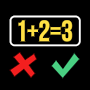# Math IQ test + Brain Training APK

1.0 for Android 4.1 and up

## Quiz & Math Game For Adults. Easy Brain Training Puzzle & Fun Mathematics Game.

Package name: com.ccbrothers.coolmathematics

Are you interested in knowing how well your brain solves mathematical puzzles and formulas?

Math IQ Test really test your mathematical skills. Math IQ Test contains several puzzle tests and speed tests and same time train your brain to solve mathematical formulas. Math IQ Test contains many different kind of math puzzle games which give you best brain training and fun time with math problems. All included mathematical IQ games and puzzles are great for adults, but easy enough for kids too.

Math IQ Test will challenge your mind in different kind of areas, you can need fast thinking, logical thinking and quick reflex. Also fast fingers required in one game.
Are you ready challenge yourself with mathematical formulas like additions, subtraction and multiplication. Game will teach and training you solve easy math problems quicker
than before. Educate your brains to work better.

Exercise your mathematical skills and become faster math solver. Everyone needs to solve mathematical problems in everyday life.

Math IQ (true or false)
Your goal is to check if mathematical formula is correct or not. You have limited time to solve every formula. Wrong answer decrease your time, correct one give you more time.

Puzzle IQ (puzzle game)
Fill the mathematical formulas with correct numbers and mathematical signs. These contains additions, subtractions and multiplications.

Cross IQ (puzzle game)
Correct mathematical formulas switching numbers.

Speed IQ (true of false)
You have 60 seconds to solve mathematical formulas. These contains additions, subtractions and multiplications.

Battle IQ (quick thinking)
Compete against your friend. The person who answers the fastest gets a point but if your answer is wrong then the opponent gets a point.

Memory IQ
Test your memory with this color pattern puzzle. How complicated patterns you can remember.

Tap IQ (fast fingers)
You have 10 seconds to tap to screen. How many times you can tap the screen?

Path IQ (puzzle game)
Fill the line with one touch. Your goal is to connect all the blocks with one touch.

Rotate IQ (puzzle game)
Rotate 3x3 areas and make a correct color pattern. This is really challenging logical puzzle game.

Letter IQ (search game)
Find the incorrect letter from the screen. Easy find and seek game.
What's New
Added more IQ games.

Category:

Date published: Sep 29, 2018

Current version: 1.0

Requires android: 4.1

Content rating: Teen

3.6
3 total
5 2
4 0
3 0
2 0
1 1